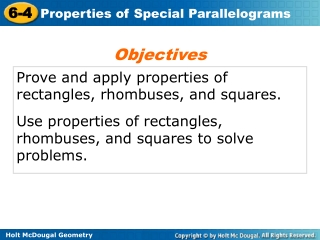DownloadDownload PresentationProve and apply properties of rectangles, rhombuses, and squares.

# Prove and apply properties of rectangles, rhombuses, and squares.

Télécharger la présentation## Prove and apply properties of rectangles, rhombuses, and squares.

- - - - - - - - - - - - - - - - - - - - - - - - - - - E N D - - - - - - - - - - - - - - - - - - - - - - - - - - -
##### Presentation Transcript

1. Objectives Prove and apply properties of rectangles, rhombuses, and squares. Use properties of rectangles, rhombuses, and squares to solve problems.

2. A second type of special quadrilateral is a rectangle. ______________– a quadrilateral with four right angles.

3. Since a rectangle is a parallelogram by Theorem 6-4-1, a rectangle “inherits” all the properties of parallelograms that you learned in Lesson 6-2.

4. Example 1: Craft Application A woodworker constructs a rectangular picture frame so that JK = 50 cm and JL = 86 cm. Find HM.

5. A rhombus is another special quadrilateral. A ______________– a quadrilateral with four congruent sides.

6. Like a rectangle, a rhombus is a parallelogram. So you can apply the properties of parallelograms to rhombuses.

7. Example 2A: Using Properties of Rhombuses to Find Measures TVWX is a rhombus. Find TV.

8. Example 2B: Using Properties of Rhombuses to Find Measures TVWX is a rhombus. Find mVTZ.

9. Helpful Hint Rectangles, rhombuses, and squares are sometimes referred to as special parallelograms. ____________ – a quadrilateral with four right angles and four congruent sides.

10. Example 3: Verifying Properties of Squares Show that the diagonals of square EFGH are congruent perpendicular bisectors of each other.

11. Step 1 Show that EG and FH are congruent. Example 3 Continued

12. Step 2 Show that EG and FH are perpendicular. Example 3 Continued

13. Step 3 Show that EG and FH are bisect each other. Example 3 Continued

14. Example 4 Given: PQTS is a rhombus with diagonal Prove:

15. Check It Out! Example 4 Continued 1. 1. 2. 2. 3. 3. 4. 4. 5. 5. 6. 6. 7. 7.